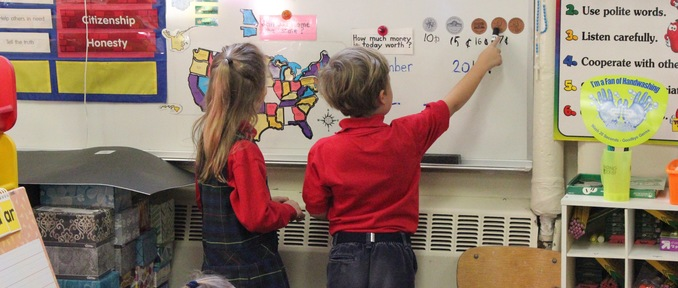# Mathematics

•The St. Joseph’s School mathematics curriculum is designed to develop mathematical proficiency through the mastery of basic skills and concepts.  Through investigation, analysis, critical thinking, collaboration and use of technology, St. Joe’s students become effective problem solvers.  Students develop the ability to effectively communicate their understanding of mathematical skills, concepts and processes.

Our math curriculum is based on a traditional approach that provides students with a strong foundation for understanding more complex concepts as they grow.  We focus on the essential concepts at each grade level to build in-depth understanding of major mathematical ideas.

Our elementary students explore mathematical processes, number operations and relationships, geometry, measurement, statistics and probability and algebraic relationships.  Our teachers use a wide variety of tools, hands-on activities and daily practice to build each student’s number sense and mental math skills and to ensure each student’s preparedness for middle school math.